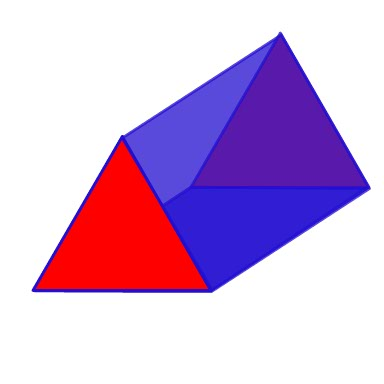## How to Calculate the Volume, Base Area and Height of a Prism | The Calculator EncyclopediaThe image above represents a prism.
To compute the volume of a prism requires two essential parameters which are the base area and height of the prism.

The formula for computing the volume of a prism is:

V = Ah

Where:
V = Volume of the Prism
A = Base area of the Prism
h = Height of the prism

Let’s solve an example
Find the volume of a prism with a base area of 30 cm2 and a height of 8 cm.

This implies that:
A = base area of the prism = 30
h= = height of the prism = 8

V = Ah
V = 30 x 8
V = 240

Therefore, the volume of the prism is 240 cm3.

Calculating the Base area of a prism when Volume and Height are Given

The formula is A = V / h

Where;
V = Volume of the Prism
A = Base area of the Prism
h = Height of the Prism

Let’s solve an example:
Find the base area of a prism with a volume of 300 cm3 and a height of 12 cm.

This implies that;
V = Volume of the prism = 300 cm3
h  = height of the prism = 12 cm

A =  V / h
A = 300 / 12
A = 25

Therefore, the base area of the prism is 25 cm2.

Calculating the height of a prism when Volume and Base area are Given

The formula is h = V / A

Where;
A = Base area of the Prism
V = Volume of the Prism
h = Height of the Prism

Let’s solve an example:
Find the height of a prism with a volume of  280 cm3  and a base area of 35 cm2

This implies that;
V = Volume of the prism = 280 cm3
A = Base area of the prism = 35 cm2

h =  V / A
h = 280 / 35
h = 8

Therefore, the height of the prism is 8 cm.